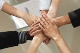# GridCalculated column question

GridTop achievementsRank 1
Kathy asked on 14 Nov 2010, 06:04 PM
I have 3 GridNumericColumns and 2 GridCalculated columns.  For the 1st calculated column, it is the product of GridNumericColumn1 and GridNumericColumn2.  What I need for the second GridCalculated column is to take the product of GridNumericColumn1 and GridNumericColumn2 and multitply that by 1 minus the value that is in GridNumericColumn3.  So if GridNumeric1 was 500 and GridNumeric2 was 10 and GridNumeric3 was 0.80, the calculation would be (500)*(10)*(1-0.8).  When I put the value 1 in the expression (1-0.8), it thinks I'm referring to column1, but I want this to be the number 1.  How would I go about doing this?  Thanks.

## 2 Answers, 1 is accepted

0Princy
Top achievementsRank 1
answered on 15 Nov 2010, 10:26 AM
Hello Kathy,

I have tried the similar scenario and it is working for me. Check whether you have set the Expression in GridCalculatedColumnas like below.

ASPX:
`<telerik:GridCalculatedColumn HeaderText=``"Total"` `DataFields=``"value1,value2,value3"`
`      ``Expression=``"{0}*{1}*(1-{2})"` `UniqueName=``"GridCalculatedColumn"``>`
`</telerik:GridCalculatedColumn>`

Thanks,
Princy.
0Kathy
Top achievementsRank 1
answered on 15 Nov 2010, 02:45 PM
Yes, that did the trick.  Thanks for your help.
Tags
GridKathy
Top achievementsRank 1Rank 1Rank 1